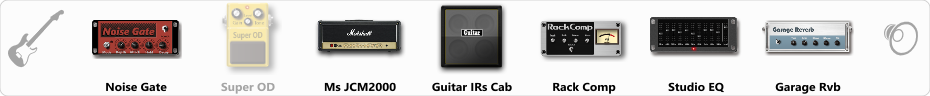# Punk #4

Discussion in 'ToneLib-GFX presets' started by Omega Station, Aug 11, 2019.

1. Punk #4

Preset name: OmSt Punk 4

You can find all the presets I made here: https://honestampsimreviews.com/preset-vault/ (under guitar amp sims - Tonelib GFX)

Effects chain:Effect: "Noise Gate" (Dynamics / Filter), active - "yes"
"Detect" = Gtr In
"Mode" = Manual
"Depth" = 50
"Threshold" = 61
"Attack" = 0
"Hold" = 5
"Decay" = 250

Effect: "Super OD" (Overdrive / Distortion), active - "no"
"Drive" = 28
"Tone" = 71
"Level" = 55

Effect: "Ms JCM2000" (Amp simulators), active - "yes"
"Gain" = 30
"Bass" = 36
"Middle" = 73
"Treble" = 74
"Presence" = 62
"Master" = 50
"Output" = 50
"Level (dB)" = 0

Effect: "Guitar IRs Cab" (Cabinets), active - "yes"
"Model" = Marshall JCM2000 (4x12")
"Mic Position" = Edge
"Mic Distance" = Near
"Low Cut (Hz)" = 95
"Hi Cut (kHz)" = 10.0
"Mix" = 100
"Level (dB)" = 0

Effect: "Rack Comp" (Dynamics / Filter), active - "yes"
"Threshold (dB)" = -20
"Ratio" = 5
"Attack" = Fast
"Release (ms)" = 250
"Knee" = 25
"Level (dB)" = -1

Effect: "Studio EQ" (Dynamics / Filter), active - "yes"
"31 Hz" = -5
"62 Hz" = -5
"125 Hz" = -4
"250 Hz" = -1
"500 Hz" = 2
"1 kHz" = 2
"2 kHz" = 1
"4 kHz" = -1
"8 kHz" = -1
"16 kHz" = -2
"above 16 kHz" = 0
"Level (dB)" = 0

Effect: "Garage Rvb" (Reverb), active - "yes"
"Time" = 5.2
"PreDelay" = 46
"LoDamp" = 11
"HiDamp" = 30
"Mix" = 10

Note: You will need to download and install the ToneLib-GFX software to use the preset.

File size:
492 bytes
Views:
3,050
File size:
492 bytes
Views:
24
File size:
705 bytes
Views:
86